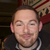0

# How do I make a timer?

Franch 7 years ago updated by icahill (Administrator) 7 years ago
Any suggestions on the best way to create a timer? I need to be able to start/stop it and also to add or subtract time from it. Right now I am trying to use a delayed loop but that doesn't seem to be getting very far.

Thanks.You should consider using javascript to accomplish this. This would involve using a [js:] (same as [eval:]) tag to evaluate.

Check out the documentation here:
https://studio.rarewire.com/wordpress/2012/05/eval...
https://studio.rarewire.com/wordpress/2013/05/java...

Javascript around timing:
http://www.w3schools.com/js/js_timing.asp+1
Here is a short example:

```           var startTime = 0;
var stopTime  = 0;

function timerStart() {
startTime =  new Date().getTime();
return startTime;

}

function timerStop() {
stopTime = new Date().getTime() - startTime;
return stopTime;
}

function formatTime() {

var time = stopTime;
var hr  = 0;
var min = 0;
var sec = 0;
var ms = 0;

hr = Math.floor( time / (60 * 60 * 1000) );
time = time % (60 * 60 * 1000);
min = Math.floor( time / (60 * 1000) );
time = time % (60 * 1000);
sec = Math.floor( time / 1000 );
ms = time % 1000;
return "" + hr + " hours " + min + " min " + sec + " sec";
}
```

Now use this in your WIRE:
```<wire>
<class name="field">
<text name="charCount" alias="FIELD1" width="50%" height="fit" color="#ffffff" font="Avenir-Medium" size="16" bottomof="text" align="center" alignment="center" text=""></text>
</class>
<main>
<panel name="2" alias="PAGE" width="95%" height="50%" align="center" _scroll="2" _name="Page One of Five" onscrollto="addStory-scroll">
<panel name="text" width="100%" height="85%" >
<panel name="1" width="100" height="100" background="ff0000" align="left" onclickup="start">
<text name="start" width="100%" height="100%" color="#ffffff" font="Avenir-Medium" size="16" alignment="center" text="Start"></text>
</panel>
<panel name="2" width="100" height="100" background="ff0000" align="right" onclickup="stop">
<text name="stop" width="100%" height="100%" color="#ffffff" font="Avenir-Medium" size="16" alignment="center" text="Stop"></text>
</panel>
</panel>
<text name="charCount" alias="FIELD" width="50%" height="fit" color="#ffffff" font="Avenir-Medium" size="16" bottomof="text" align="center" alignment="center" text="Start"></text>
</panel>
</main>
<actions>
<action name="start">
<sync>
<delete target="FIELD"/>
<delete target="FIELD1"/>
<assign property="var:startTime" value="[js: timerStart(); ]"/>
<play action="timer" />
</sync>
</action>
<action name="timer">
<create class="field" target="PAGE"/>
<sync delay="1" loop="yes">
<assign property="var:stopTime" value="[js: timerStop();]"/>
<assign property="object:FIELD1.text" value="[js: formatTime(); ]" />
</sync>
</action>
<action name="stop">
<sync>
<assign property="var:startTime" value="0"/>
<delete target="FIELD1"/>